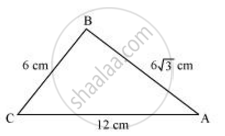Share

# Tick the correct answer and justify: In ΔABC, AB = 6√3 cm, AC = 12 cm and BC = 6 cm. The angle B is - CBSE Class 10 - Mathematics

#### Question

Tick the correct answer and justify: In ΔABC, AB = 6sqrt3 cm, AC = 12 cm and BC = 6 cm.

The angle B is:

• 120°

• 60°

• 90°

• 45°

#### SolutionGiven that, AB = 6sqrt3 cm, AC = 12 cm, and BC = 6 cm

It can be observed that

AB2 = 108

AC2 = 144

And, BC2 = 36

AB2 +BC2 = AC2

The given triangle, ΔABC, is satisfying Pythagoras theorem.

Therefore, the triangle is a right triangle, right-angled at B.

∴ ∠B = 90°

Hence, the correct answer is 90°.

Is there an error in this question or solution?

#### APPEARS IN

NCERT Solution for Mathematics Textbook for Class 10 (2019 to Current)
Chapter 6: Triangles
Ex. 6.50 | Q: 17 | Page no. 151

#### Video TutorialsVIEW ALL 

Solution Tick the correct answer and justify: In ΔABC, AB = 6√3 cm, AC = 12 cm and BC = 6 cm. The angle B is Concept: Pythagoras Theorem.
S